# 10+ Multiplying Fractions Worksheet Templates – PDF

Fraction multiplication is a crucial part of secondary school maths curriculum. The students are needed to practice hard on the fraction multiplications as these form the basis of many maths problems later on. Thus, there are multiplying fractions worksheets to help the students with good practice. If you are a maths teacher, you are often required to create these worksheets for your entire class. Keeping in mind your busy schedule, creating a worksheet seems like quite a tedious affair. But not to worry any more as these days you have the sample Multiplying fractions worksheets templates online.

## Multiplication of Fractions Worksheets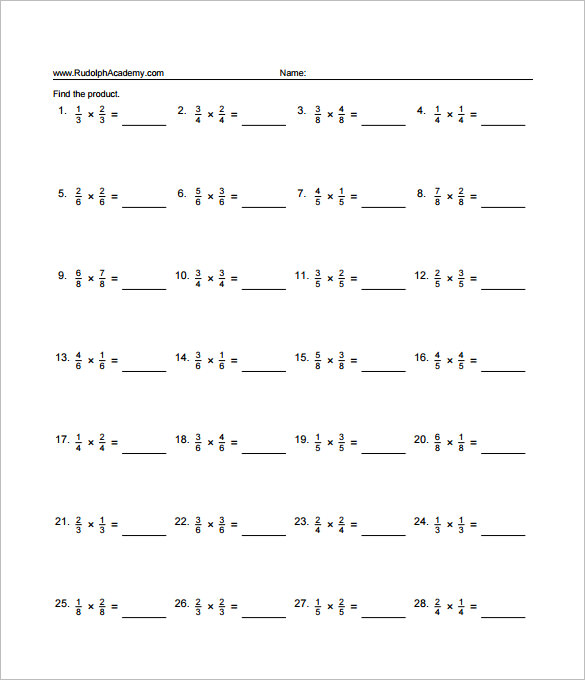## Multiplying Fractions Printable Worksheets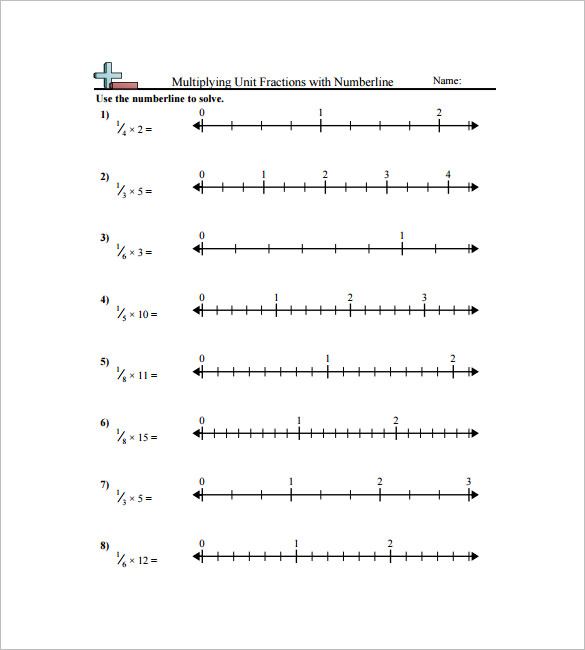commoncoresheets.com

## Editable Multiplying Fractions Worksheet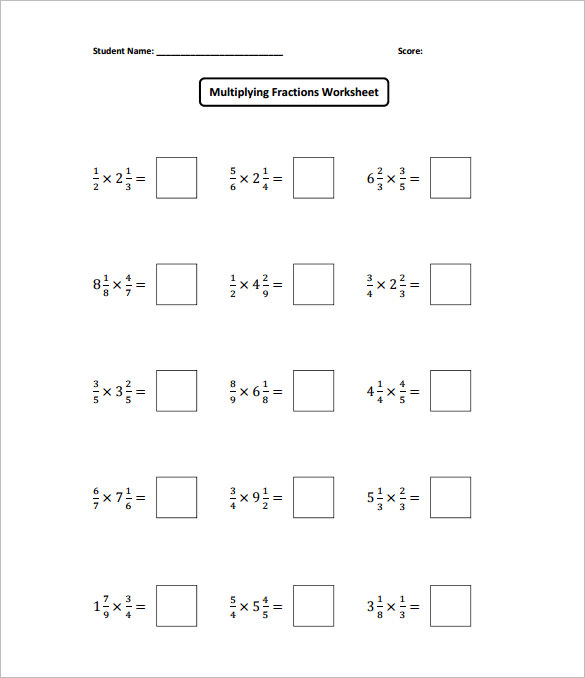mathworksheets4kids.com

## Multiplying Fractions Worksheet KS 2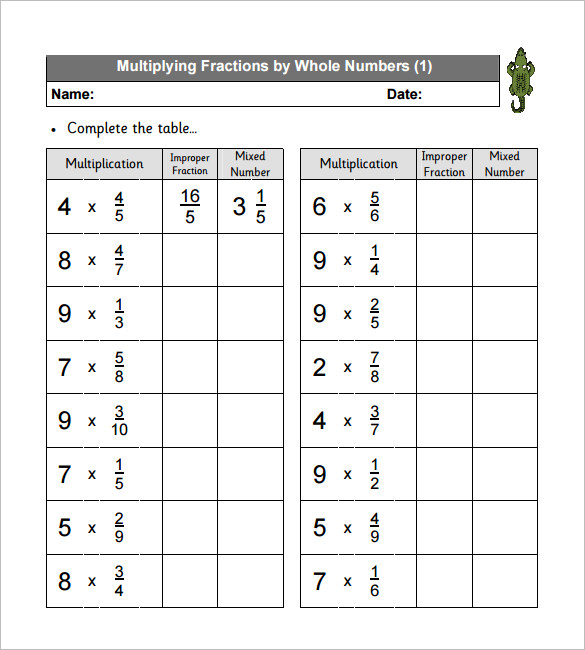snappymaths.com

## Multiplying Fractions Worksheets 5th Grade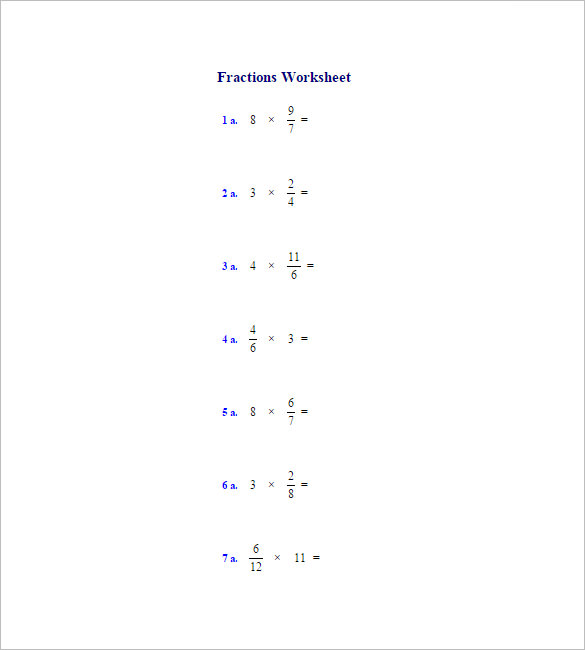homeschoolmath.net

## Multiplying Fractions Worksheets 6th Grade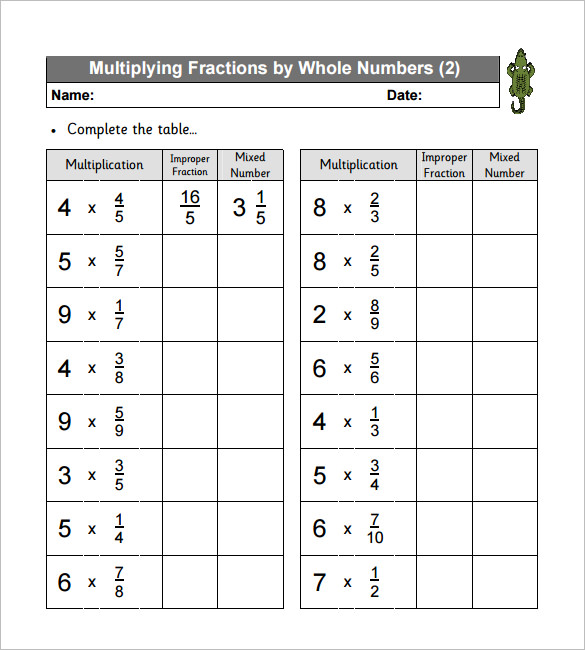## Multiplying Fractions Worksheets KS 3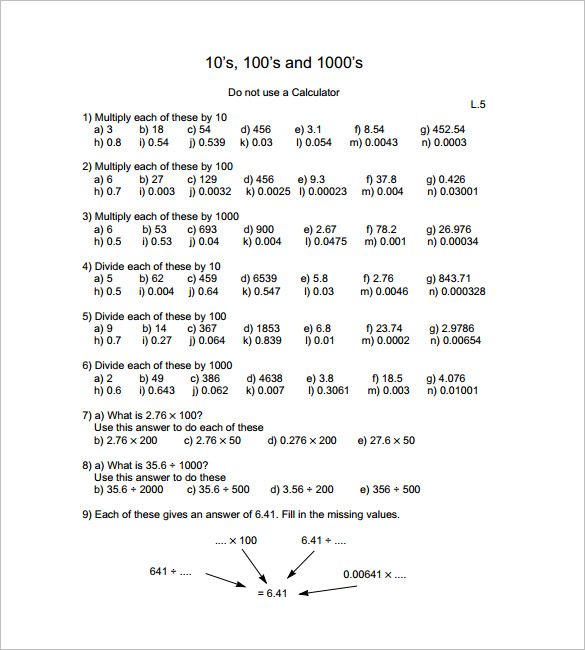m4ths.com

## Multiplying Fractions Worksheets With Answers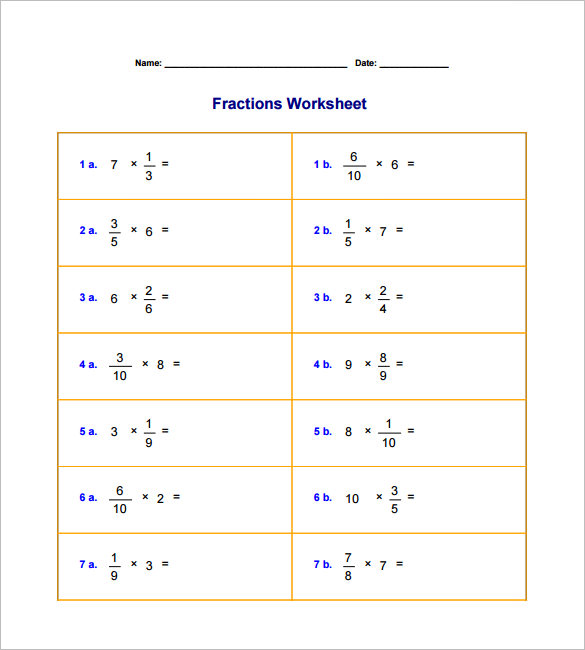homeschoolmath.net

## Cross Multiplying Fractions Worksheets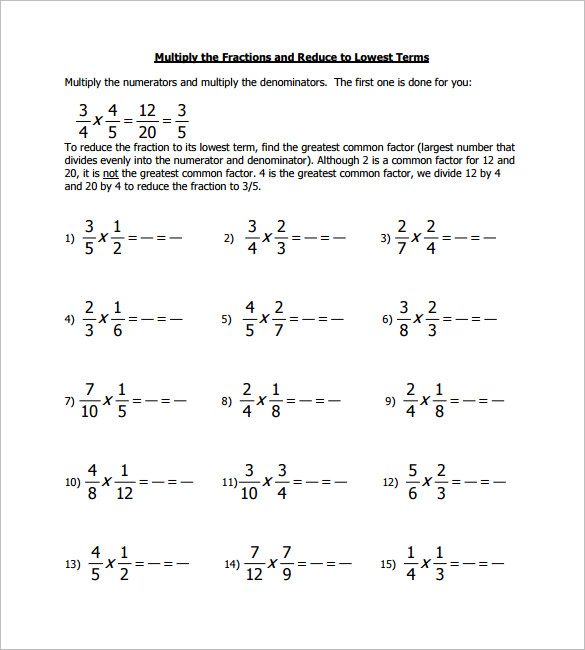calvarychristianschool.net

## Sample Fraction Multiplication Worksheet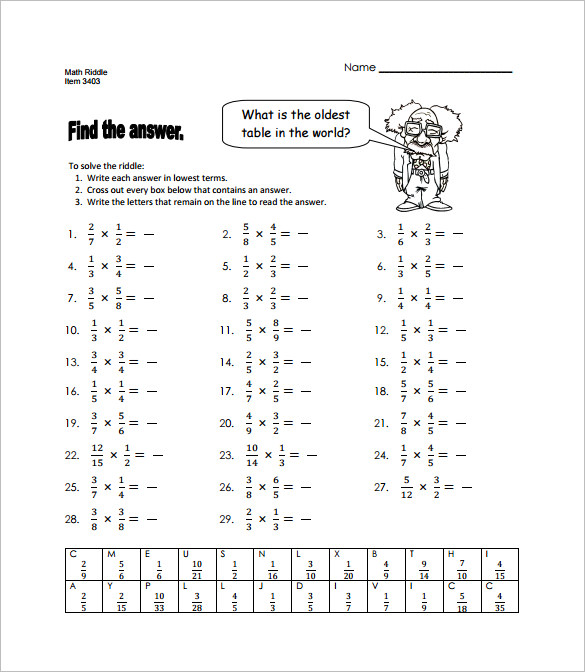tlsbooks.com

## Multiplying Fractions Questions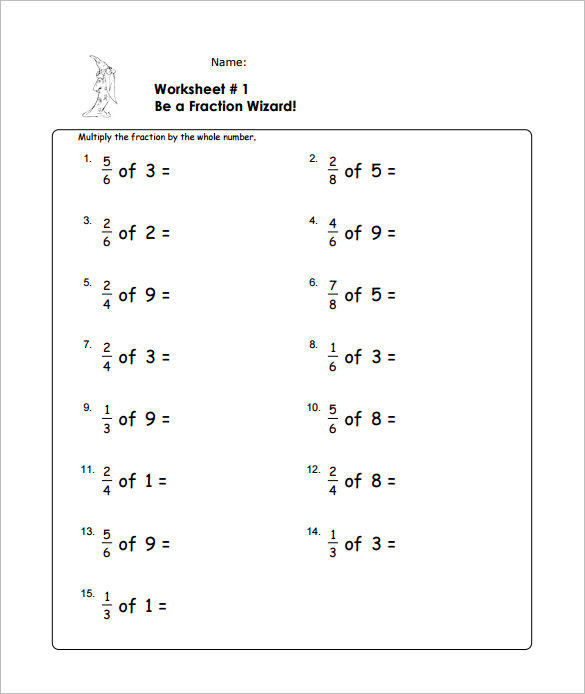mathworksheets4kids.com

## Benefits of these templates

• These worksheet templates feature a ready made worksheet where you have the problem fractions already mentioned. You would just need to download, print and pass on the worksheets to the students.
• You are relieved from the entire hassle of creating a whole new worksheet from scratch.
• These online worksheets, are, thus great time & energy savers for you.
• You can use the same worksheet for every student in your entire class.
• The online world offers a wide range of these worksheets in different levels of complexities.
• Many sites offer free Multiplying fractions worksheets.